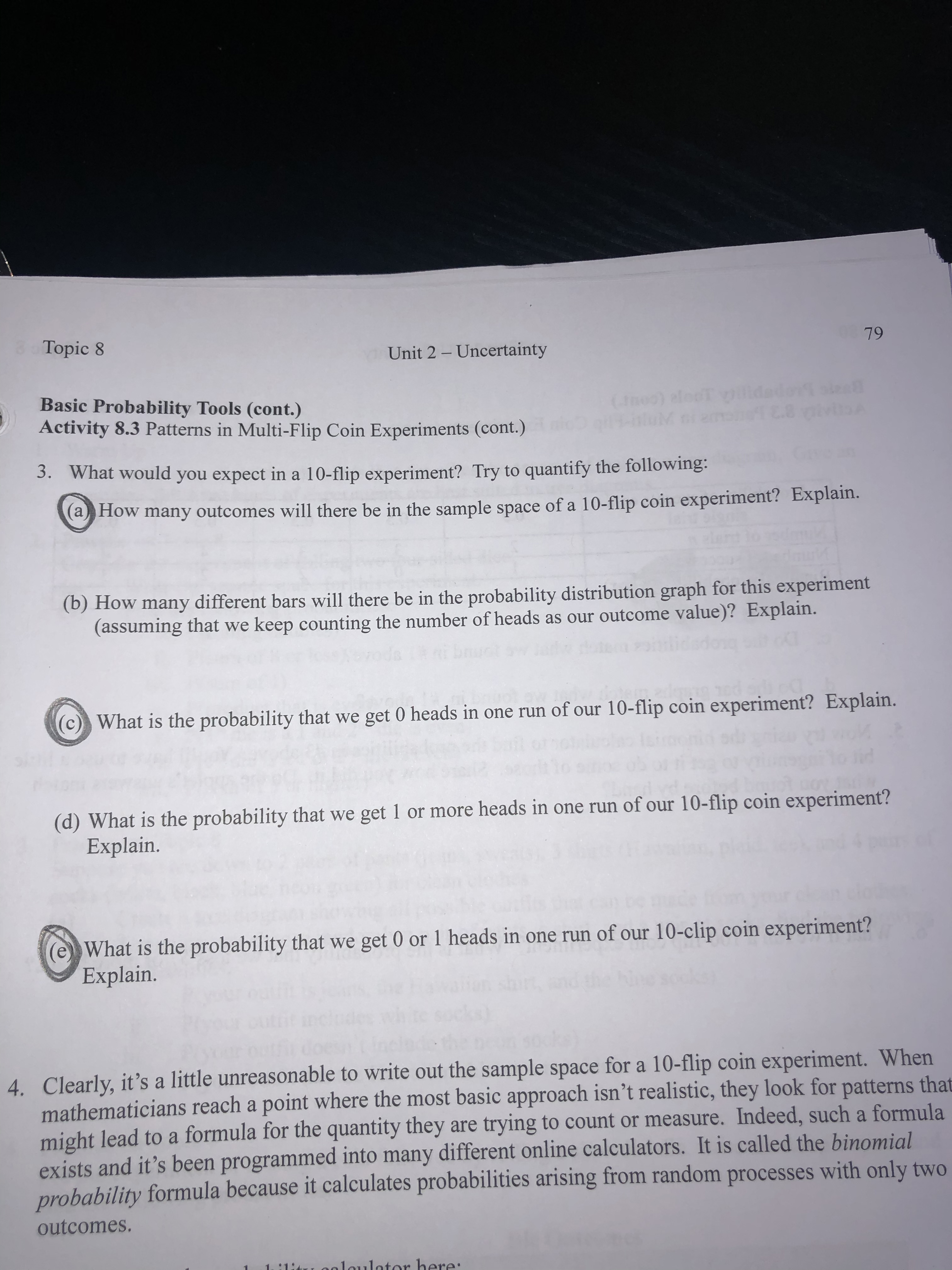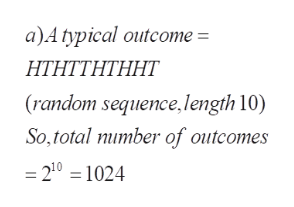Topic 879Unit 2 - UncertaintyBasic Probability Tools (cont.)Activity 8.3 Patterns in Multi-Flip Coin Experiments (cont.)(oc) alod ulded2 yI3. What would you expect in a 10-flip experiment? Try to quantify the following(aHow many outcomes will there be in the sample space of a 10-flip coin experiment? Explain.(b) How many different bars will there be in the probability distribution graph for this experiment(assuming that wekeep counting the number of heads as our outcome value)? Explain.(c) What is the probability that we get 0 heads in one run of our 10-flip coin experiment? Explain.(d) WhatExplain.the probability that we get 1 or more heads in one run of our 10-flip coin experiment?What is the probability that we get 0 or 1 heads in one run of our 10-clip coin experiment?Explain.4. Clearly, it's a little unreasonable to write out the sample space for a 10-flip coin experiment. Whenmathematicians reach a point where the most basic approach isn't realistic, they look for patterns thatmight lead to a formula for the quantity they are trying to count or measure. Indeed, such a formulaexists and it's been programmed into many different online calculators. It is called the binomialprobability formula because it calculates probabilities arising from random processes with only twooutcomes.:12t aoult r re:

Questionhelp_outlineImage TranscriptioncloseTopic 8 79 Unit 2 - Uncertainty Basic Probability Tools (cont.) Activity 8.3 Patterns in Multi-Flip Coin Experiments (cont.) (oc) alod ulded 2 yI 3. What would you expect in a 10-flip experiment? Try to quantify the following (aHow many outcomes will there be in the sample space of a 10-flip coin experiment? Explain. (b) How many different bars will there be in the probability distribution graph for this experiment (assuming that we keep counting the number of heads as our outcome value)? Explain. (c) What is the probability that we get 0 heads in one run of our 10-flip coin experiment? Explain. (d) What Explain. the probability that we get 1 or more heads in one run of our 10-flip coin experiment? What is the probability that we get 0 or 1 heads in one run of our 10-clip coin experiment? Explain. 4. Clearly, it's a little unreasonable to write out the sample space for a 10-flip coin experiment. When mathematicians reach a point where the most basic approach isn't realistic, they look for patterns that might lead to a formula for the quantity they are trying to count or measure. Indeed, such a formula exists and it's been programmed into many different online calculators. It is called the binomial probability formula because it calculates probabilities arising from random processes with only two outcomes. :12t aoult r re: fullscreen
Step 1

Problems related to probability of events when 10 coins are tossed (flipped) , independently

Step 2

a) We may represent each of the 10 tosses by H (head) orT (tail). So each experiment can be described as a sequence of H or T (randomly) of length 10. So the total number of outcomes is 2^10=1024. (as each of the 10 places can be filled in 2 ways )help_outlineImage Transcriptionclosea)A typical outcome = ΗΤΗΤΤΗΤΗΗΤ (random sequence,length 10) So, total number of outcomes = 210 = 1024 fullscreen
Step 3

(b) Denote the probability of Head as c; then the probability of 0-heads in a 10 -f...

Want to see the full answer?

See Solution

Want to see this answer and more?

Our solutions are written by experts, many with advanced degrees, and available 24/7

See Solution
Tagged in

Basic Probability